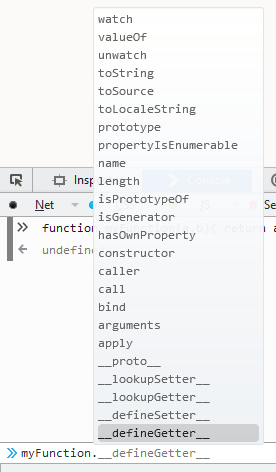# Javascript - Function Expression (Anonymous function)

A Function Expression is just an function in the form of an expression stored generally in a variable that you execute by adding its signature ([param,[, param,[…, param]]])

A function expression is not created in the global context.

A function expression is a convenient way for creating closure. See Closure example

## Named function expression

### Recursive expression

A named function expressions binds its name as a local variable within the function and allows so to write recursive function expressions.

Example with a count mathematical function.

``````var foo = function rec(v) {
if (v <= 1) {
v = 1;
} else {
v += " + "+rec((v-1));
}
return v;
}
mathExpression = foo(5);
console.log(mathExpression+ " = " + eval(mathExpression));```
```

### Debugging

The name of the function expressions will be found in the stack traces.

## Example

### Expression stored in a variable

The below example binds the function to a variable 'add' rather than myOptionalName.

``````var add = function myOptionalName(x, y) {
return x + y;
};

```

where:

• the function name (myOptionalName) is optional and default to _variableName (in this case add) inside the function (if recursivity is needed)

### Expression directly used

The above can also be rewritten as a Immediately invoked function expression (IIFE).

``````console.log(
/* Parentheses means execute: here the function expression */
(  function (x, y) { return x + y; } ) (2,3) /* Pass the variables 2 and 3 */
)```
```

Recommended Pages
Javascript - Immediately invoked function expression (IIFE)

An IIFE (pronounced “iffy”) is a function expression that is immediately invoked in order to create a local scope for the variables They therefore won’t affect the surrounding code outside of it...
Javascript - Arrow Function Expression

An arrow function expression has a shorter syntax than a function expression and does not bind its own: this, arguments, super, or new.target. These function expressions are best suited for...
Javascript - Callback

This page is callbacks in Javascript. It is the primary method of enabling asynchrony. With argument, you are not passing the function but the return value of the function. You need then to wrap...
Javascript - Closure

Closure in Javascript The most common usage of closure in JavaScript is the module pattern. Closures have access and can update their outer variables. Closures store their outer variables by references,...
Javascript - Function Declaration

A function declaration is one of the several grammar syntax that permits to define a function. The execution of a function declaration can be done before the function declaration definition. This is...Javascript - Functions

Functions functionalities in Javascript. A function in Javascript is a variable that’s given a reference to the function being declared. The function itself is a value (as an integer or an array) that...